In the circuit, the potential difference across PQ will be nearest to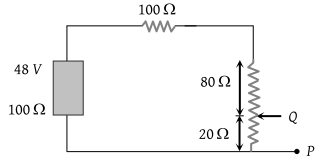(1) 9.6 V

(2) 6.6 V

(3) 4.8 V

(4) 3.2 V

Concept Questions :-

Combination of resistors
High Yielding Test Series + Question Bank - NEET 2020

Difficulty Level:

Three resistors are connected to form the sides of a triangle ABC, the resistance of the sides AB, BC and CA are 40 ohms, 60 ohms and 100 ohms respectively. The effective resistance between the points A and B in ohms will be

(1) 32

(2) 64

(3) 50

(4) 200

Concept Questions :-

Combination of resistors
High Yielding Test Series + Question Bank - NEET 2020

Difficulty Level:

Find the equivalent resistance across AB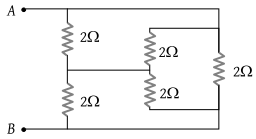(1) 1 Ω

(2) 2 Ω

(3) 3 Ω

(4) 4 Ω

Concept Questions :-

Combination of resistors
High Yielding Test Series + Question Bank - NEET 2020

Difficulty Level:

Two wires of the same dimensions but resistivities ρ1 and ρ2 are connected in series. The equivalent resistivity of the combination is

(1) ρ1 + ρ2

(2) $\frac{{\rho }_{1}+{\rho }_{2}}{2}$

(3) $\sqrt{{\rho }_{1}{\rho }_{2}}$

(4) $2\left({\rho }_{1}+{\rho }_{2}\right)$

Concept Questions :-

Combination of resistors
High Yielding Test Series + Question Bank - NEET 2020

Difficulty Level:

A 3 volt battery with negligible internal resistance is connected in a circuit as shown in the figure. The current I, in the circuit will be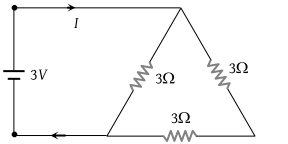(1) 1/3 A

(2) 1 A

(3) 1.5 A

(4) 2 A

Concept Questions :-

Combination of resistors
High Yielding Test Series + Question Bank - NEET 2020

Difficulty Level:

Find the equivalent resistance between the points a and b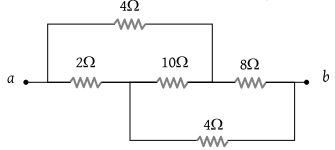(1) 2 Ω

(2) 4 Ω

(3) 8 Ω

(4) 16 Ω

Concept Questions :-

Wheatstone bridge
High Yielding Test Series + Question Bank - NEET 2020

Difficulty Level:

The potential difference between point A & B is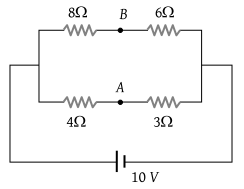(1) $\frac{20}{7}\text{\hspace{0.17em}\hspace{0.17em}}V$

(2) $\frac{40}{7}\text{\hspace{0.17em}\hspace{0.17em}}V$

(3) $\frac{10}{7}\text{\hspace{0.17em}\hspace{0.17em}}V$

(4) 0

Concept Questions :-

Wheatstone bridge
High Yielding Test Series + Question Bank - NEET 2020

Difficulty Level:

In the circuit shown below, The reading of the voltmeter V is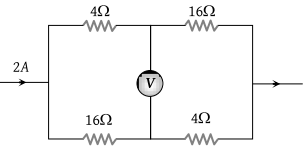(1) 12 V

(2) 8 V

(3) 20 V

(4) 16 V

Concept Questions :-

Wheatstone bridge
High Yielding Test Series + Question Bank - NEET 2020

Difficulty Level:

In the circuit shown in the adjoining figure, the current between B and D is zero, the unknown resistance is of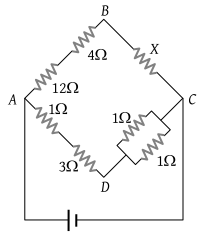(1) 4 Ω

(2) 2 Ω

(3) 3 Ω

(4) em.f. of a cell is required to find the value of X

Concept Questions :-

Wheatstone bridge
High Yielding Test Series + Question Bank - NEET 2020

Difficulty Level:

In the circuit shown in the figure, the current flowing in 2 Ω resistance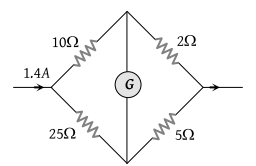(1) 1.4 A

(2) 1.2 A

(3) 0.4 A

(4) 1.0 A

Concept Questions :-

Wheatstone bridge